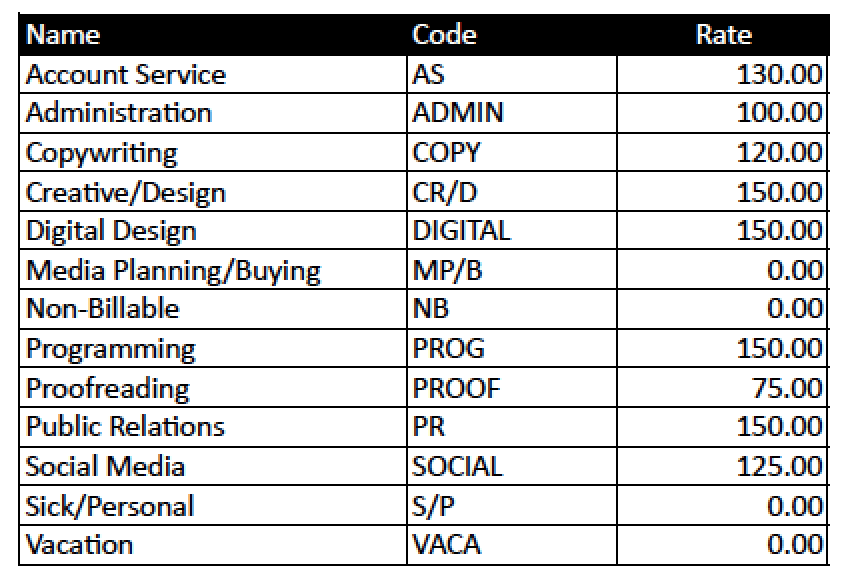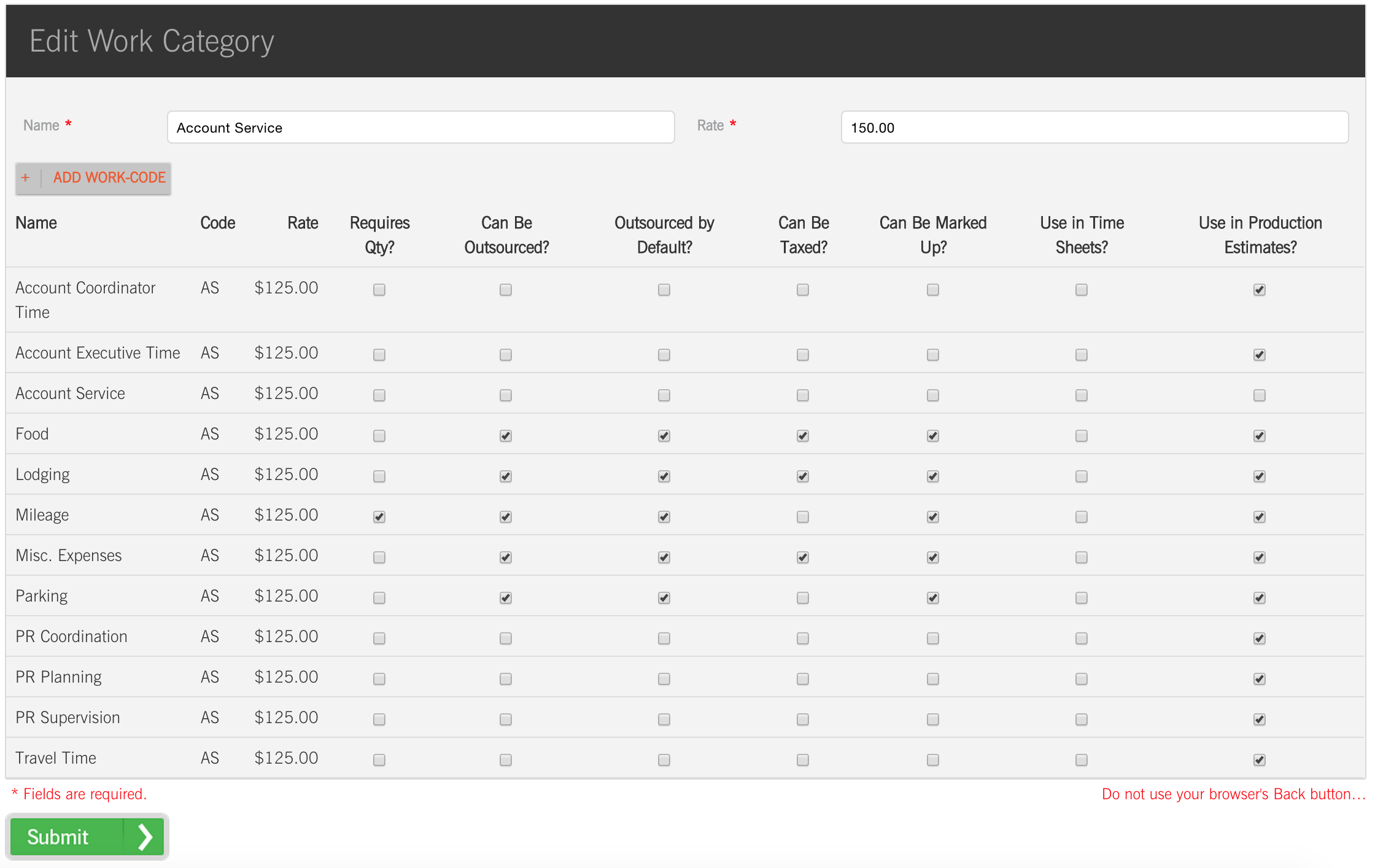## Adding (and Managing) Work Codes

CONVENTIONS

QuickTIPs (and Links) are highlighted in blue.

Permission-based items are highlighted in red.

Work Codes, by their very nature, are somewhat complicated. CurrentTrack® recommends breaking the work of your agency down into blended Categories with easy-to-understand abbreviations and up-to-date hourly rates. For example:TIP: If Employees bill at different hourly rates, create separate Work Codes for each (e.g. Tom = 100.00 and Allison = 125.00). When they record time, they can do so under their own Codes. The Rates associated with each will only be displayed within the Production Estimate form and [job] Financial Report, not within the Time Sheet drop-down menu.

Other Work Codes to consider are Principal, Account Planning, Art Direction, Production, Project Management, Estimating, Research, Strategic Planning, SEO/SEM Facilitation, and Web Content Development. The number of Codes included in CurrentTrack depends upon how detailed your agency is in its time tracking. An unlimited number of Categories and Work Codes can be entered. They flow through CurrentTrack, enabling some of its most useful features such as the Production Estimate, so take care to enter and edit them correctly.

To create a Work Code, go to Admin — System Administration — Manage Work Codes.

A Work Code must be filed beneath a parent Work Category. If the applicable Work Category already exists (e.g. Account Service), simply click on its name and then Add Work Code. If the parent Work Category does not yet exist, click Add Work Category. Next, enter the Name and Rate of the Category (e.g. Account Service at a Rate of 150.00); click Submit.

Next, create a Work Code beneath the new Category by going to Work Category (name) — Add Work Code.

Enter a Name (e.g. Account Service), Code (e.g. AS) and Rate (e.g. 150.00). Don’t worry about the length of the Name. The field is unlimited and CurrentTrack will automatically create a Key at the bottom of the edit Time Sheet screen. As elsewhere in CurrentTrack, avoid using comma + number combinations (e.g. 1,000.00) in the Rate field.

Next, determine where you’d like the Code to appear within CurrentTrack. If you’d like it to appear in the Time Sheet Code drop-down menu, for example, check the box beneath Use in Time Sheets. If you’d like it to appear in your Production Estimate form, check the box beneath Use in Production Estimates. If the Code will be used in the Production Estimate form, check the corresponding boxes beneath Requires Qty, Can be Outsourced, Outsourced by Default, Can be Taxed, and Can be Marked Up; click Submit.

TIP: A good rule of thumb when creating Production Estimate Work Codes is, if you’ve assigned it a Qty. field, it’ll also need an Outsourced box. The equation CurrentTrack will use is as follows: Qty. x Outsourced (yes) x Cost = Net Cost + Tax and/or Markup = Subtotal.

Any number of Work Codes can be added beneath a Work Category. For example:TIP: If you’re adding a Work Code, and it’s typically freelanced, assign both Qty. and Outsourced boxes. You can then enter the number of Hours spent on the Task (e.g. Copywriting) in its Qty. field (e.g. 3 [Hours]). If the Task is done in-house, no Qty. or Outsourced boxes are necessary. Simply enter the number in the Hours/Cost field (e.g. 3 [Hours of Copywriting]).

CHANGING A WORK CODE RATE

In order to change a Work Code Rate (e.g. Account Service from 150.00 to 200.00), you’ll need to first create a new Work Code that reflects the change(s) by going to Work Category (name) — Add Work Code.

Next, deactivate the old Work Code by going to Work Category — [click] Name. Locate the desired Work Code line and un-check the boxes beneath Use in Time Sheet and Use In Production Estimate, as well as any other check-marked criteria; Submit.

Existing Rates cannot simply be overwritten. You wouldn’t want to change the dollar figures on a Production Estimate you’d already given to the Client or on a Time Sheet that had already been recorded, for example.

ARCHIVING A WORK CODE

To archive a Work Code, go to Admin — System Administration — I Want To: Manage Work Codes — [click on] Work Category [Name]. Locate the desired Work Code line and un-check the boxes beneath Use in Time Sheet and Use In Production Estimate, as well as any other check-marked criteria; Submit.

SCENARIO

Which Work Codes do you recommend be included in the Production Estimate form?

We recommend including the following Work Codes in your form. These items have been listed under the corresponding Work Categories that are typically used in an advertising agency. It’s entirely up to you, of course, as to which Categories and Codes you ultimately incorporate into your system.

 Account Service (Code: AS)(Rate: \$130.00/hr.) Item Qty. Outsourced Hours/Cost Net Cost Tax Markup Subtotal Account Coordinator Time X X X Account Executive Time X X X Food X X X X X X Lodging X X X X X X Mileage X X X X X X Miscellaneous Expenses X X X X X X Parking X X X X X PR Coordination X X X PR Planning X X X PR Supervision X X X Travel Time X X X Administration (Code: A)(Rate: \$100.00/hr.) Item Qty. Outsourced Hours/Cost Net Cost Tax Markup Subtotal Catering X X X X X X Delivery Charges X X X X X Entertainment X X X X X X Legal Services X X X X X Miscellaneous Expenses X X X X X X Postage X X X X X Printing Estimation X X X Printing Supervision X X X Project Research X X X Proofreading X X X Rush Charges X X X X Systems Support X X X X Telephone Charges X X X X X Creative (Code: C)(Rate: \$150.00/hr.) Item Qty. Outsourced Hours/Cost Net Cost Tax Markup Subtotal 3-D Animation X X X X X Agency Fees X X X X Art Direction X X X Artwork Disk(s) X X X X X X X Audio X X X X X X Broadcast Director X X X B/W Lasers X X X X X X X Camera/Crew X X X X X Casting X X X X X Color Outputs X X X X X X X Concept Development X X X Copywriting X X X X X X Creative Direction X X X Film Stock X X X X X X Graphics X X X X X X Grip X X X X X Illustration X X X X X Lights X X X X X X Location Fees X X X X X X Location Shoot Time X X X X Make-up X X X X X Master Cost X X X X X X Miscellaneous Art Expenses X X X X X X Miscellaneous Broadcast Expenses X X X X X X Miscellaneous PR Expenses X X X X X X Music X X X X X X Photography X X X X X X Photography Director X X X X Press Clipping X X X Production Company X X X X X X Product Styling X X X X X X Props X X X X X X Retouching X X X Rights/Fees X X X X X Scans X X X X X X X Set Design/Construction X X X X Stock Photos X X X X X X Sytlist X X X X X Talent X X X X X Type Formatting X X X Velox(es) X X X X X X X Video Stock X X X X X X Voiceover(s) X X X X X X Wardrobe X X X X X X Digital (Code: D)(Rate: \$150.00/hr.) Item Qty. Outsourced Hours/Cost Net Cost Tax Markup Subtotal Audio Dubs X X X X X X X Audio Mix X X X X CD Dubs X X X X X X X CD Packaging X X X Film Transfer/Processing X X X X X X HTML Tweaking X X X Interactive CD Design X X X Internet Planning/Design X X X Internet Programming/Debugging X X X X X Miscellaneous Digital Expenses X X X X X X Motion Design/FX/Compositing X X X X Offline Video Editing X X X X Online Video Editing X X X X Sample Production X X X X Special Effects X X X X Video Dubs X X X X X X X Media (Code: M)(Rate: \$0.00/hr.) Item Qty. Outsourced Hours/Cost Net Cost Tax Markup Subtotal Billable Planning/Buying Time X X X Media Fees X X X X X X Media Research X X X X Miscellaneous Media Expenses X X X X X X Post Analysis Time X X X PR Newswire Service X X X X